• 总体参数用什么表示
千次阅读
2020-12-06 17:58:50

简单统计推断：总体参数的假设检验
1.假设检验的逻辑步骤
（1）写出零假设和备择假设
（2）确定检验统计量
（3）确定显著性水平α
（4）根据数据计算检验统计量的实现值
（5）得到检验是否显著的结论

2.对于正态总体均值的检验
2.1根据一个样本对其总体均值大小进行检验
单尾检验
双尾检验【如果一个单尾检验问题用了双尾检验，p值就比单尾检验时大了一倍】

2.2根据来自两个总体的独立样本对其总体均值的检验

w=read.table('drug.txt',header=T) #读入数据
x=w[w[,2]==1,1];y=w[w[,2]==2,1]   #分开两个数据
t.test(x,y,alt='greater')         #检验【不检验方差，直接用方差不等的方法去做，不会有问题】


2.3成对样本的问题

t.test(w$before,w$after,alt='greater',pair=T)    #直接检验
t.test(w$before-w$after,alt='greater')           #相减后检验


2.4关于正态性检验的问题
(1)最简单实用方法：Shapiro正态性检验
(2)直观办法:正态QQ图（不一定正确）:用样本分位数与正态分位数做散点图，如果总体是正态的，则图上的点应该近似地排成一条直线。

x=scan('sugar.txt')
qqnorm(x);qqline(x)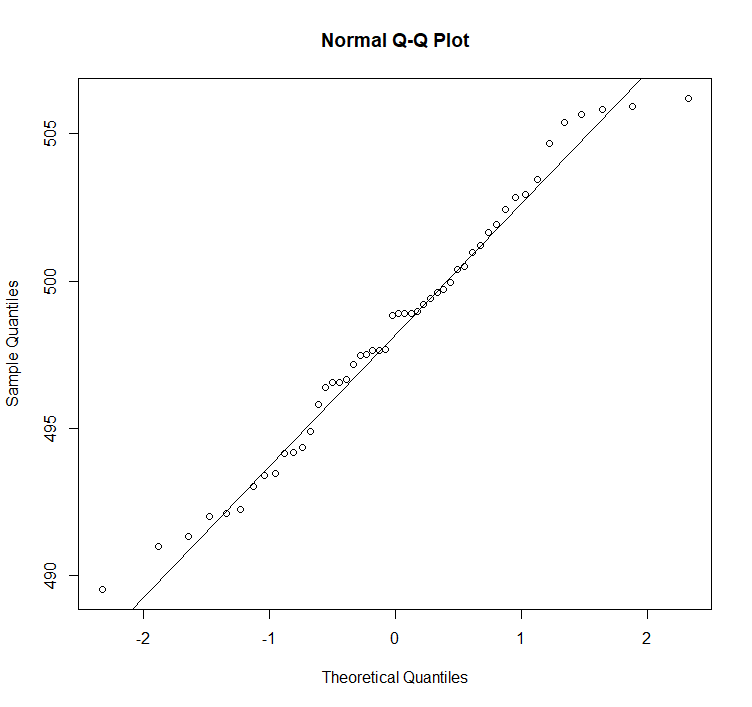3.对于比例的检验
3.1对于总体比例的检验
(1)精确检验【首选】

binom.test(0.23*1500,1500,.25,alt='less')


两个总体比例之差p1-p2的检验：

binom.test(c(.2*1200,.21*1300),c(1200,1300),alt='less')


(2)利用公式的近似检验【计算机不发达时的遗产】
(3)用连续性修正的近似检验

3.2对于连续变量比例的检验
两种等价形式检验：
1.看中位数或α分位数是否是某个事先认定的值（零假设）
2.大于（或小于）某数的观测值是否为一个事先认定的比例（零假设）
Q:存活时间低于2小时的是否少于70%？【此检验又称为（推广的）符号检验，不用对总体分布进行任何假定，通常把符号检验归于非参数检验范畴】

x=scan('life.txt')
binom.test(sum(x<2),60,.7,alter='greater')


4.非参数检验
4.1关于非参数检验的一些常识
（1）和数据本身的总体分布无关的检验称为非参数检验。
（2）在总体分布已知时，传统方法有较大的势，效率要高【势（power）：当备择假设正确时，该检验拒绝零假设的概率，强势检验也称为高效率检验】 但在总体分布未知时，非参数统计比假定了错误总体分布时的传统方法要高，有时要高很多。
（3）如何比较检验的效率：通常用两种检验方法的渐近相对效率（ARE）来度量，当ARE等于1时表示两者效率一样。一般来说，检验T1对检验T2的相对效率是这两个检验在拒绝零假设时所使用的最小样本量n1和n2的反比：n2/n1，显然，用的样本量越少，效率越高。
（4）秩（rank）的概念：一般来说，秩就是该数据按照升幂排列之后，每个观测值的位置。

4.2关于单样本位置的符号检验【3.2有介绍广义的符号检验】
狭义的符号检验

x=scan('gs.txt')
pbinom(sum(x>100),25,.5)


大于零假设中位数m的个数等于所有观测值减去m之后所得的符号为正的差的个数，而小于m的个数等于符号为负的差个数。这就是之所以称为符号检验的原因。
如果有等于中位数m的，即既不相应于属于正号，又不相应于属于负号，对判断没有贡献，一般把它删除。

4.3关于单样本位置的Wilcoxon符号秩检验
要求假定样本点来自连续对称总体分布，对总体中位数的检验，等价于对总体均值的检验。
该检验的具体步骤：
(1)对i=1,…,n，计算∣Xi-M0∣，它们代表这些样本点到M0的距离。
(2)把上面的n个绝对值排序，并找出它们的n个秩，如果它们有相同的样本点，每个点取平均秩（如1,4,4,5的秩为1,2.5,2.5,4）。
(3)令W+等于Xi-M0>0的∣Xi-M0∣的秩的和，而W-等于Xi-M0<0的∣Xi-M0∣的秩的和。
(4)对双边检验H0：M=M0<=>H1：M≠M0，在零假设下，W+和W-应差不多。因而，当其中之一很小时，应怀疑零假设。在此，取检验统计量W=min(W+,W-)
(5)根据得到的W值，利用统计软件或查Wilcoxon符号秩检验的分布表以得到在零假设下的p值。如果n很大要用正态近似：得到一个与W有关的正态随机变量Z的值，再用软件或查正态分布表得到p值。
(6)如果p值较小（比如小于或等于给定的显著性水平，譬如0.05）则可以拒绝零假设。如果p值较大则没有充分的证据来拒绝零假设，但不意味着接受零假设

4.4关于随机性的游程检验（runs test）
游程检验方法：检验一个取两个值的变量的这两个值的出现是否时随机的。
游程：样本中相同的值在一起称为一个游程，单独的值也算。

library(tseries)
x=scan('run1.txt')
runs.test(factor(x))


当然，游程检验并不仅仅用于只取两个值的变量，它还可以用于某个连续变量的取值小于某个确定值及大于该值的个数（类似于0和1的个数）是否随机的问题。

library(tseries)
x=scan('run2.txt')
runs.test(factor(x>median(x))) #这里runs.test()不是精确检验


4.5比较两独立总体中位数的Wilcoxon（Mann-Whitney)秩和检验
检验所需唯一假定：两个总体的分布有类似形状（不一定对称)
原理：假定第一个样本有m个观测值，第二个样本有n个观测值，把两个样本混合之后把这m+n个观测值按照大小次序排序，然后记下每个观测值在混合排序下面的秩。之后分别把两个样本所得到的秩相加，记秩和分别是Xx和Xy，这两个值可以互相推算，称为Wilcoxon统计量。该统计量的分布与两个总体分布无关，由此分布可以得到p值。

w=read.table('gdp.txt')
wilcox.test(w[w[,2]==1,1],w[w[,2]==2,1],alt='less') #单尾检验r语言
更多相关内容
• 本文介绍总体参数 总体包含所有研究样本，总体参数即是描述总体分布的参数，如总体均值、标准差，【机器学习中训练集得到的模型可以理解为一种总体参数】。 实际研究中，由于时间和经济的原因，我们几乎无法获取所有...

本文介绍总体参数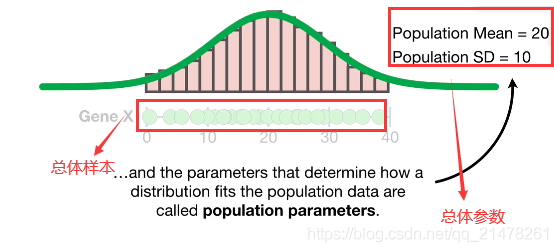总体包含所有研究样本，总体参数即是描述总体分布的参数，如总体均值、标准差，【机器学习中训练集得到的模型可以理解为一种总体参数】。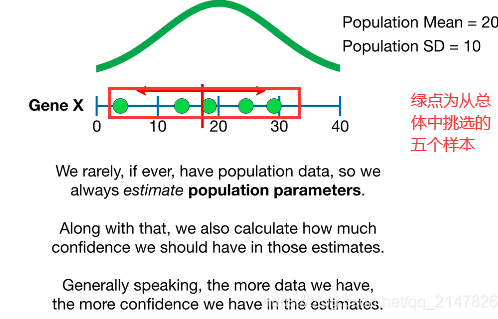实际研究中，由于时间和经济的原因，我们几乎无法获取所有总体数据，所以总体参数是无法直接计算的，只能通过部分总体样本估计获得

同时计算估计值的置信度，获取的数据越多，置信度越高（置信度后续会介绍），估计的总体的参数越可能是正确。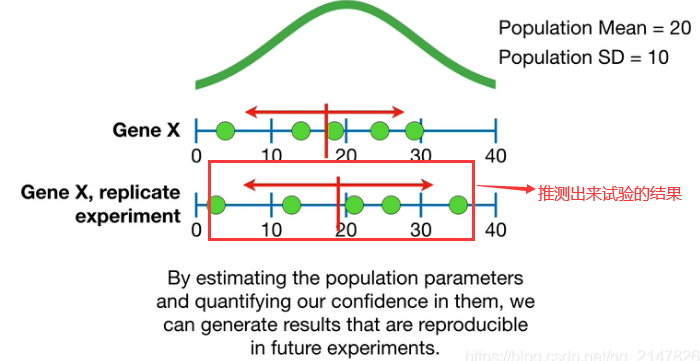通过估计总体参数并量化其置信度，我们就可以在将来的试验中产生可以重复的结果推测将来试验的结果

# Reference

https://www.bilibili.com/video/BV1iJ411R7zf?p=4展开全文• 推断统计研究的是如何根据样本数据去推测总体特征的方法。进行推断的原因是在实际生产中，获取总体数据通常比较困难，甚至不可能完成，因此需要对总体进行抽样，通过样本统计量去估计总体参数

本文总结了数据分析中用到的推断统计分析知识点，是自己学习参数估计和假设检验的学习笔记。

推断统计研究的是如何根据样本数据去推测总体特征的方法。进行推断的原因是在实际生产中，获取总体数据通常比较困难，甚至不可能完成，因此需要对总体进行抽样，通过样本统计量去估计总体参数。

# 一、参数估计

## 1. 点估计

就是用样本的统计量去代替总体参数。
优点： 可以给出具体的估计值；
缺点： 估计结果容易受到随机抽样的影响，无法保证结论的准确性。

## 2. 区间估计

根据样本统计量计算出一个可能的区间与概率。
优点： 可给出合理的范围（置信区间）及信心指数（置信度）；
缺点： 不能给出具体的估计值。

### 2.1 中心极限定理

如果总体（分布不重要）均值为 μ方差为 σ 2 σ^2 ，进行足够多次随机抽样，样本容量为n，当n增大时，样本均值近似服从正太分布X~N(μ， σ 2 σ^2 /n)。
结论：
1、多次抽样，每次抽样会得到一个均值，均值会围绕在总体均值左右，呈正太分布。
2、当样本容量n足够大（一般n>=30），样本均值服从正太分布。
1）样本均值构成的正态分布，其均值等于总体均值μ；
2）样本均值构成的正态分布，其标准差等于σ/ n \sqrt n
注意：
1、样本均值的标准差，称为标准误差，简称标准误
2、区分总体标准差σ，样本标准差（一次抽样获得的标准差），标准误差（样本均值构成的正太分布的标准差）。

### 2.2 程序模拟中心极限定理

import numpy as np
import pandas as pd
import seaborn as sns

#定义总体数据
#loc：均值；scale：标准差；size：数组大小，即数组中含有的元素个数
all_ = np.random.normal(loc=30,scale=80,size=10000)
#创建均值数组，用来存放每次抽样（每个样本）的均值
mean_arr = np.zeros(1000)
for i in range(len(mean_arr)):
#随机抽样，size：样本容量；replace：是否为放回抽样，默认为True
mean_arr[i] = np.random.choice(all_,size=64,replace=False).mean()
#样本均值构成正态分布，该正态分布的均值等于总体均值
#标准差等于总体标准差/根号n
print('样本均值构成的正太分布的均值：',mean_arr.mean())
print('样本均值构成的正太分布的标准差（标准误）：',mean_arr.std())
print('偏度：',pd.Series(mean_arr).skew())
sns.distplot(mean_arr)


样本均值构成的正太分布的均值： 30.653383960104826
样本均值构成的正太分布的标准差（标准误）： 9.918944517394578
偏度： 0.05852723907161612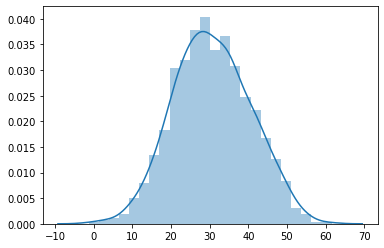### 2.3 正态分布的特性

1、以均值为中心，在1倍标准差内（ μ-σ，μ+σ），包含约68%的样本数据。
2、以均值为中心，在2倍标准差内（μ-2σ，μ+2σ），包含约95%的样本数据。
3、以均值为中心，在3倍标准差内（μ-3σ，μ+3σ），包含约99.7%的样本数据。
其中，σ为样本均值的标准差，即标准误差。

### 2.4 程序检验正态分布的特性

#标准差
scale = 50
x = np.random.normal(0,scale,size=100000)
#定义标准差的倍数，倍数从1到3
for times in range(1,4):
y = x[(x > -times * scale) & (x < times * scale)]
print(times,'倍标准差：',f'{len(y) * 100 / len(x)}%')


1 倍标准差： 68.392%
2 倍标准差： 95.499%
3 倍标准差： 99.734%

### 2.5 行业应用

参数估计在行业的应用，具体操作要根据实际场景来：
1、工业生产量的判断。
2、服务业投诉量是否改进判断。
3、电商业用户访问量、用户流失等评估。
4、金融、保险业风险评估。

# 二、 假设检验

区间估计是通过正面方式，来计算总体参数的可能取值（区间）；而假设检验是从反正角度来判断，是接受原假设还是拒绝原假设。

## 1. 相关概念

1、小概率事件
小概率事件在一次试验中不会发生，一旦发生则拒绝原假设。
接受原假设，并不代表原假设一定是正确的，只是没有充分的证据去证明原假设是错误的，因此只能接受原假设。
2、P-Value与显著性水平
P-Value是支持原假设的概率，设定的阈值（α表示）为显著性水平，α通常选定为0.1、0.05、0.01，具体根据样本量选择，常选0.05。
3、原假设与备择假设
1）若是等值估计，等值选为原假设，不等选为备择假设；
2）在单边检验中，原假设为维持现状，改变现状为备择假设。

## 2.假设检验的步骤

1、设置原假设和备择假设；
2、设置显著性水平α（通常选择0.05）；
3、根据问题选择检验方式；
4、计算统计量，并通过统计量获取P值；
5、根据P值与α值，决定接受原假设还是备择假设。

## 3. Z检验

Z检验用来判断样本均值与总体均值是否有显著性差异。Z检验是通过正太分布理论来推断差异发生的概率，从而比较两个均值的差异是否显著。

### 3.1 适用场景

1、总体呈正态分布；
2、总体方差已知；
3、样本容量较大（一般>=30）
1和3满足其一即可，因为在样本容量很大时，样本均值近似呈正态分布。

### 3.2 Z统计量计算方式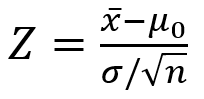x一把：样本均值；
μ0：待检测的总体均值；
σ：总体的标准差
n：样本容量
Z统计量含义： 样本均值与总体均值的距离是几倍标准误差，如果大于1.96倍或小于-1.96倍，表示样本均值落在了拒绝域。

### 3.3 Z检验Python实现

from scipy import stats
已知：样本 a = np.array([])，总体均值mean，总体标准差std
样本均值：sample_mean = a.mean()
标准误差：se = std / np.sqrt(len(a))
Z统计量：Z = (sample_mean = mean) / se
P值：P = 2 * stats.morm.sf(abs(Z))


## 4. t 检验

t检验用来判断样本均值是否与总体均值具有显著性差异，t检验是基于t分布的。

### 4.1 适用场景

1、总体呈正态分布；
2、总体方差未知；
3、样本数量较少（<30）
当样本容量>30，t分布接近正太分布

### 4.2 t 统计量计算方式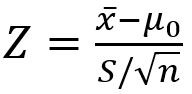x一把：样本均值；
μ0：待检测的总体均值；
S：样本的标准差
n：样本容量

### 4.3 t 检验Python实现

已知：样本 a = np.array([])，总体均值mean
样本均值：sample_mean = a.mean()
样本标准差：sample_std = a.std()
t统计量：(sample_mean - mean) / (sample_std / np.sqrt(len(a)))
P值：2 * stats.t.sf(abs(t),df=len(a)-1)，df为自由度，


简单理解，自由度就是变量能够自由取值的个数，t分布的自由度为n-1

展开全文• 用来估计总体参数的统计量称为估计量，θ^\hat{\theta}θ^表示。样本均值、样本比例、样本方差都可以是一个估计量。 估计值 根据一个具体的样本计算出来的估计量的数值，称为估计值。比如样本量计算出来的平均...

本章内容：

1. 相关专业名词解释
2. 如何估计一个总体参数的范围，及如何选择对应的公式？
3. 如何估计两个总体参数的范围，及如何选择对应的公式？
4. 如何确定总体估计时需要的样本量？

# 7.1 涉及的专业名词解释

### 7.1.1 估计量与估计值_名词解释

📌 参数估计
用样本统计量去估计总体的参数。

📌 估计量
用来估计总体参数的统计量称为估计量，用 θ ^ \hat{\theta} 表示。样本均值、样本比例、样本方差都可以是一个估计量。

📌 估计值
根据一个具体的样本计算出来的估计量的数值，称为估计值。比如用样本量计算出来的平均值作为总体的平均值，那么这个平均值在这时就称为估计值。

### 7.1.2 点估计与区间估计

💡 excel中计算指定概率对应的面积公式：=normsinv(指定的概率)
例：当显著性为5%时， Z α / 2 = n o r m s i n v ( 1 − 5 % 2 ) = n o r m s i n v ( 0.975 ) Z_{\alpha/2}=normsinv(1-\frac{5\%}{2})=normsinv(0.975)

📌 点估计

用样本统计量 θ ^ \hat{\theta} 的某个取值直接作为总体参数 θ \theta 的估计值。比如用样本均值 x ˉ \bar{x} ，作为总体均值 μ \mu 的估计值。

📖 在重复抽样条件下，点估计的均值可望等于总体真值。

由于样本是随机的，抽出一个具体的样本得到的估计值很可能不同于总体真值，所以在使用点估计代表总体参数值时，需要给出点估计值的可靠性，即说明点估计值与总体参数的真实值的接近程度。

由于点估计值的可靠性由抽样标准误差衡量，所以具体的点估计值无法给出估计可靠性的度量，故需要围绕点估计值构造总体参数的一个区间，这是区间估计。

📌 区间估计
给出总体参数估计的一个区间范围，该区间通常由样本统计量加减估计误差得到。

📖 与点估计不同，区间估计时，根据样本统计量的抽样分布可以对样本统计量与总体参数的接近程度给出一个概率度量。

📌 置信区间
样本统计量所构成的总体参数的估计区间称为置信区间，其中区间的最小值称为置信下限，最大值称为置信上限。

可以理解为假设在需要估计GMV，估计的正确率需要达到95%，在95%的概率下计算出来GMV处于[100,101]，得出的这个区间就是置信区间。

📌 置信水平
置信区间中包含总体参数真值的次数所占的比例称为置信水平，也称为置信度，表示为 1 − α 1-\alpha ，其中 α \alpha 表示错误率，也称为显著性水平。

可以理解为参数估计的正确率，如上述GMV例子中的95%。

📖 样本量、置信水平与置信区间的关系
当样本量给定时，置信区间的宽度随着置信水平的增大而增大；
当置信水平固定时，置信区间的宽度随着样本量的增大而减小，即较大的样本所提供的有关总体的信息更多。

📖 对置信区间的理解，有3点需注意：

1. 怎么理解置信水平为95%的置信区间？
如果用某种方法构造的所有区间中有95%的区间包含总体样本的真值，5%的区间不包含总体参数的真值，那么用该方法构造的区间称为置信水平为95%的置信区间。

2. 置信区间会因为样本不同而不同；
总体参数的真值是固定的、未知的，而样本构造的区间是不固定的。所以置信区间是一个随机区间，会因为样本的不同而不同，而且不是所有的区间都包含总体参数的真值。

3. 置信水平是针对随机区域而言的；
不是用来描述某个特定区间包含总体参数真值的可能性。

比如某班级学生平均考试成绩置信水平为95%的置信区间为[60,80]，不能说60~80分以95%的概率包含全班学生平均考试的真值。我们只知道在多次抽样中，95%的样本得到的区间包含全班学生平均考试成绩的真值。

### 7.1.3 评价估计量的标准

📌 无偏性

样本期望与总体参数无偏，即估计量抽样分布的数学期望等于被估计量总体参数；
设总体参数为 θ \theta ，样本的估计量为 θ ^ \hat{\theta} ，如果 E ( θ ^ ) = θ E(\hat{\theta})=\theta ，则称 θ ^ \hat{\theta} θ \theta 的无偏估计。

当样本均值的期望值等于总体均值，样本比例的期望值等于总体比例，那么样本方差的期望值等于总体误差。

📌 有效性
更小标准差的估计量更有效；对同一总体参数的两个无偏估计量，有更小标准差的估计量更有效。

📌 一致性
估计量与总体一致；随着样本量的增大，估计量的值越来越接近被估总体的参数，即样本量越大，标准差应该越小

# 7.2 如何估计一个总体参数的范围，及如何选择对应的分布公式？

总体思路：
根据样本和总体数据集的情况，以及需要求的参数是什么，来选择不同的分布公式。将指标带入公式计算，则得到总体的参数估计量。

比如：想通过样本数据集，求总体的均值是多少。如果样本数据集是大样本，则选择Z分布的公式；如果是小样本，则需要看总体的方差是否已知，如果总体方差不可得，则选择t分布的公式。

☑️ 对不同的参数进行估计，对应选择的不同分布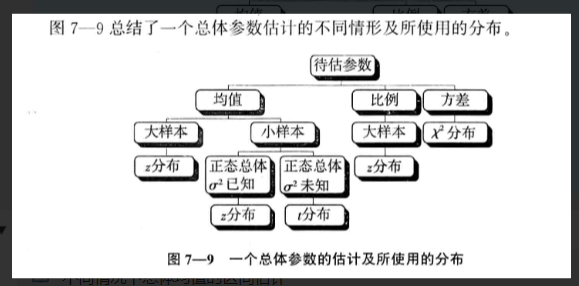### 7.2.1 如何对总体均值的区间进行估计？

📖 不同情况下总体均值的区间估计公式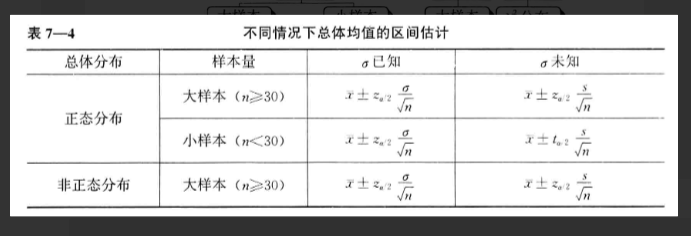💡 z分布区间计算的excel公式：

=avg(样本值)±normsinv(1-$\alpha/ 2 ) ∗ 标 准 差 / 2)*标准差/ \sqrt{样本数量}$

估计总体均值，等于样本均值±给定显著性水平下的面积*标准差/样本数量开平方。即样本均值±均值估计误差

💡 excel中，t分布的临界值公式：=tinv(a,df)，其中a为对应与双尾t分布的概率，df为自由度。
例如求 t α / 2 t_{\alpha/2} ，其中a=0.05，则公式为：=tinv(0.05,20)

☑️ 总体均值区间估计的考虑因素：

• 总体是否为正态分布
• 总体方差是否已知
• 估计量的样本是大样本（n≥30）还是小样本（n<30）

情况一：📖 正态分布， σ 2 \sigma^2 已知；总体不是正态分布，大样本

样本均值 x ˉ \bar{x} 的抽样分布均为正态分布， E ( x ˉ ) = μ E(\bar{x})=\mu D ( x ˉ ) = σ 2 n D(\bar{x})=\frac{\sigma^2}{n} ，样本经过标准化以后的随机变量服从标准正态分布：

z = x ˉ − μ σ / n ∼ N ( 0 , 1 ) z=\frac{\bar{x}-\mu}{\sigma/\sqrt{n}}\sim{N(0,1)}

根据以上条件，再加上正态分布的性质，可以得出总体均值 μ \mu 1 − α 1-\alpha 置信水平下的置信区间为：

x ˉ ± z α / 2 σ n \bar{x}\pm z_{\alpha/2}\frac{\sigma}{\sqrt{n}}

α \alpha 称为显著性水平，也就是错误率；
1 − α 1-\alpha 称为置信水平；
z α / 2 z_{\alpha/2} 是当显著性水平为 α / 2 \alpha/2 时的总体面积；
σ n \frac{\sigma}{\sqrt{n}} 是总体标准误差；
z α / 2 σ n z_{\alpha/2}\frac{\sigma}{\sqrt{n}} 是估计总体均值时的估计误差；

情况二：📖 正态分布， ∗ ∗ σ 2 **\sigma^2 未知；总体不是正态分布，大样本**

样本均值 x ˉ \bar{x} 的抽样分布均为正态分布， E ( x ˉ ) = μ E(\bar{x})=\mu ，使用样本方差 s 2 s^2 代替总体方差 σ 2 \sigma^2 ，样本经过标准化以后的随机变量服从标准正态分布：

z = x ˉ − μ s / n ∼ N ( 0 , 1 ) z=\frac{\bar{x}-\mu}{s/\sqrt{n}}\sim{N(0,1)}

根据以上条件，再加上正态分布的性质，可以得出总体均值 μ \mu 1 − α 1-\alpha 置信水平下的置信区间为：

x ˉ ± z α / 2 s n \bar{x}\pm z_{\alpha/2}\frac{s}{\sqrt{n}}

α \alpha 称为显著性水平，也就是错误率；
1 − α 1-\alpha 称为置信水平；
z α / 2 s n z_{\alpha/2}\frac{s}{\sqrt{n}} 是估计总体均值时的估计误差；

情况三：📖 正态分布， σ 2 \sigma^2 未知，小样本

样本均值 x ˉ \bar{x} 的抽样分布均为正态分布， E ( x ˉ ) = μ E(\bar{x})=\mu ，样本均值经过标准化以后的随机变量则服从自由度为（n-1）的t分布：

t = x ˉ − μ s / n ∼ t ( n − 1 ) t=\frac{\bar{x}-\mu}{s/\sqrt{n}}\sim{t(n-1)}

根据以上条件，再加上正态分布的性质，可以得出总体均值 μ \mu 1 − α 1-\alpha 置信水平下的置信区间为：

x ˉ ± t α / 2 s n \bar{x}\pm t_{\alpha/2}\frac{s}{\sqrt{n}}

α \alpha 称为显著性水平，也就是错误率；
1 − α 1-\alpha 称为置信水平；
t α / 2 t_{\alpha/2} 是自由度为（n-1）时，t分布中右侧面积为 α / 2 \alpha/2 时的t值；

📖 t分布
类似正态分布的一种对称分布，通常比正态分布平坦和分散，一个特定的t分布依赖于称之为自由度的参数。随着自由度的增大，t分布逐渐趋于正态分布。

### 7.2.2 如何对总体比例的区间进行估计？

📖 本章内容的前提条件：
此节只讨论大样本情况的总体比例的估计问题。

对于总体比例的估计，确定样本是否足够大的一般经验规则是：
区间 p ± 2 p ( 1 − p ) / 2 p\pm2\sqrt{p(1-p)/2} 中不包含0或1，或者要求np≥5和n(1-p)≥5

🔑总体比例 π \pi 已知时：
由样本比例p的抽样分布可知，当样本量足够大时，样本比例p的抽样分布可用正态分布近似。p的数学期望为 E ( p ) = π E(p)=\pi ；p的方差为 σ p 2 = π ( 1 − π ) n \sigma_p^2=\frac{\pi(1-\pi)}{n}

样本比例经标准化后的随机变量服从标准正态分布，公式如下：

z = p − π π ( 1 − π ) / n ∼ N ( 0 , 1 ) z=\frac{p-\pi}{\sqrt{\pi(1-\pi)/n}}\sim{N(0,1)}

总体比例 π \pi 1 − α 1-\alpha 置信水平下的置信区间为：

p ± z α / 2 π ( 1 − π ) n p\pm z_{\alpha/2}\sqrt{\frac{\pi(1-\pi)}{n}}

🔑 总体比例 π \pi 未知时：
如果 π \pi 未知，需要用样本比例p来代替 π \pi ，这时置信区间为：

p ± z α / 2 p ( 1 − p ) n p\pm z_{\alpha/2}\sqrt{\frac{p(1-p)}{n}}

🗣 案例：估算总体比例的置信区间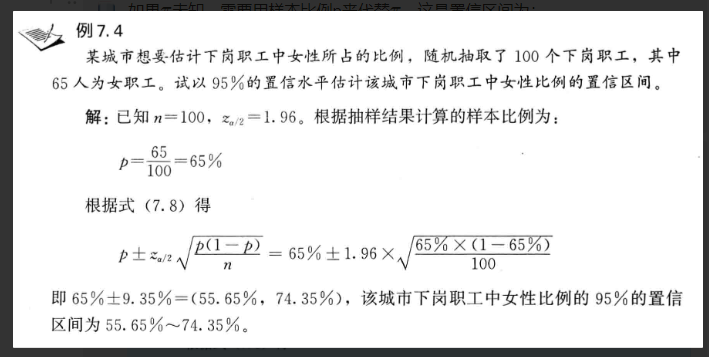### 7.2.3 如何对总体方差的区间进行估计？

📖 本节内容前提条件
此节只讨论正态总体方差的估计问题。

📖 excel中， χ 2 \chi^2 分布概率计算公式为：=chiinv(概率，自由度)

📖 χ 2 \chi^2 分布与总体方差区间估计公式

根据样本方差的抽样分布可知，样本方差服从自由度为n-1的 χ 2 \chi^2 分布。

在给定显著性水平 α \alpha χ 2 \chi^2 分布构造的总体方差 σ 2 \sigma^2 的置信区间如下图所示：由于 ( n − 1 ) s 2 σ 2 ∼ χ 2 ( n − 1 ) \frac{(n-1)s^2}{\sigma^2}\sim{\chi^2(n-1)} ，故可得：

χ 1 − α / 2 2 ≤ ( n − 1 ) s 2 σ 2 ≤ χ α / 2 2 \chi_{1-\alpha/2}^2\le{\frac{(n-1)s^2}{\sigma^2}}\le{\chi_{\alpha/2}^2}

从而推导出总体方差的置信区间为：

( n − 1 ) s 2 χ α / 2 2 ≤ σ 2 ≤ ( n − 1 ) s 2 χ 1 − α / 2 2 \frac{(n-1)s^2}{\chi_{\alpha/2}^2}\le{\sigma^2}\le{\frac{(n-1)s^2}{\chi_{1-\alpha/2}^2}}

# 7.3 如何估计两个总体参数的区间范围，及如何选择对应的分布公式？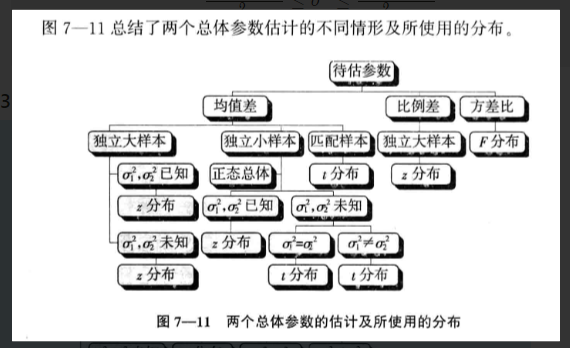### 7.3.1 如何估计两个总体的均值之差的区间范围？

📖 情况一：独立大样本时
如果两个样本是从两个总体中独立抽取，且两个总体都为正态分布；或两个总体不服从正态分布，但两个样本都是大样本（n≥30）。

其中总体均值为 μ 1 \mu_1 μ 2 \mu_2 ；样本均值为 x ˉ 1 \bar{x}_1 x ˉ 2 \bar{x}_2

那么两个样本均值之差 x ˉ 1 − x ˉ 2 \bar{x}_1-\bar{x}_2 的抽样分布服从期望值为 ( μ 1 − μ 2 ) (\mu_1-\mu_2) 、方差为 ( σ 1 2 n 1 + σ 2 2 n 2 ) (\frac{\sigma_1^2}{n_1}+\frac{\sigma_2^2}{n_2}) 的正态分布。

两个样本均值之差经标准化后服从标准正态分布，即

z = ( x ˉ 1 − x ˉ 2 ) − ( μ 1 − μ 2 ) σ 1 2 n 1 + σ 2 2 n 2 ∼ N ( 0 , 1 ) z=\frac {(\bar{x}_1-\bar{x}_2)-(\mu_1-\mu_2)} {\sqrt{\frac{\sigma_1^2}{n_1}+\frac{\sigma_2^2}{n_2}}} \sim{N(0,1)}

🔑 当两个总体的方差 σ 1 2 \sigma_1^2 σ 2 2 \sigma_2^2 已知时，两个总体均值之差在 1 − α 1-\alpha 的置信水平下，置信区间为：

( x ˉ 1 − x ˉ 2 ) ± z α / 2 σ 1 2 n 1 + σ 2 2 n 2 (\bar{x}_1-\bar{x}_2)\pm z_{\alpha/2}{\sqrt{\frac{\sigma_1^2}{n_1}+\frac{\sigma_2^2}{n_2}}}

🔑 当两个总体的方差 σ 1 2 \sigma_1^2 σ 2 2 \sigma_2^2 未知时，可用样本方差 s 1 2 s_1^2 s 2 2 s_2^2 来代替，两个总体均值之差在 1 − α 1-\alpha 的置信水平下，置信区间为：

( x ˉ 1 − x ˉ 2 ) ± z α / 2 s 1 2 n 1 + s 2 2 n 2 (\bar{x}_1-\bar{x}_2)\pm z_{\alpha/2}{\sqrt{\frac{s_1^2}{n_1}+\frac{s_2^2}{n_2}}}

📖 情况二：独立小样本时

当样本都为小样本时，需要假定：
1） 两个总体都服从正态分布
2） 两个随机样本独立地分别抽自两个总体

🔑 当两个总体方差未知但相等时，即 σ 1 2 = σ 2 2 \sigma_1^2=\sigma_2^2 ，可以将两个样本的数据组合在一起，给出总体方差的合并估计量 s p 2 s_p^2 ，公式为

s p 2 = ( n 1 − 1 ) s 1 2 + ( n 2 − 1 ) s 2 2 n 1 + n 2 − 2 s_p^2=\frac{(n_1-1)s_1^2+(n_2-1)s_2^2} {n_1+n_2-2}

这时，两个样本均值之差标准化后服从自由度为 （ n 1 + n 2 − 2 ） （n_1+n_2-2） 的t分布，公式为：

t = ( x ˉ 1 − x ˉ 2 ) − ( μ 1 − μ 2 ) s p 1 n 1 + 1 n 2 ∼ t ( n 1 + n 2 − 2 ) t=\frac{(\bar{x}_1-\bar{x}_2)-(\mu_1-\mu_2)} {s_p\sqrt{\frac{1}{n_1}+\frac{1}{n_2}}} \sim{t(n_1+n_2-2)}

两个总体均值之差在 1 − α 1-\alpha 的置信水平下的置信区间为：

( x ˉ 1 − x ˉ 2 ) ± t α / 2 ( n 1 + n 2 − 2 ) s p 2 ( 1 n 1 + 1 n 2 ) (\bar{x}_1-\bar{x}_2)\pm t_{\alpha/2}(n_1+n_2-2) \sqrt{ s_p^2(\frac{1}{n_1}+\frac{1}{n_2}) }

🔑 当两个总体方差未知且不相等时，两个样本均值之差经标准化后近似服从自由度为 v v 的t分布，自由度 v v 的计算公式为：

v = ( s 1 2 n 1 + s 2 2 n 2 ) 2 ( s 1 2 / n 1 ) 2 n 1 − 1 + ( s 2 2 / n 2 ) 2 n 2 − 1 v=\frac{ (\frac{s_1^2}{n_1} +\frac{s_2^2}{n_2})^2 }{ \frac{(s_1^2/n_1)^2}{n_1-1} +\frac{(s_2^2/n_2)^2}{n_2-1} }

两个总体均值之差在 1 − α 1-\alpha 的置信水平下的置信区间为：

( x ˉ 1 − x ˉ 2 ) ± t α / 2 ( v ) s 1 2 n 1 + s 2 2 n 2 (\bar{x}_1-\bar{x}_2)\pm t_{\alpha/2}(v) \sqrt{ \frac{s_1^2}{n_1} +\frac{s_2^2}{n_2} }

📖 情况三：匹配样本时

由于独立样本的潜在弊端，可以使用匹配样本，即一个样本中的数据与另一个样本中的数据相对应。

举例：

独立样本时，每种方法随机指派12个工人，可能会将技术较差的12个工人指定给方法1，技术较好的工人指定给方法2，这种不公平的指派可能会掩盖两种方法组装产品所需时间的真正差异。

匹配样本时，先指定12个工人用第一种方法，然后再让这12个工人用第二种方法，这样得到的数据就是匹配数据。

匹配样本可以消除由样本指定的不公平造成的两种方法组装时间上的差异。

🔑 大样本条件下，均值之差 μ d = μ 1 − μ 2 \mu_d=\mu_1-\mu_2 ，在 1 − α 1-\alpha 置信水平下的置信区间为：

d ˉ ± z α / 2 σ d n \bar{d}\pm {z_{\alpha/2}\frac{\sigma_d}{\sqrt{n}}}

d表示两个匹配样本对应数据的差值， d ˉ \bar{d} 表示各个差值的均值；
σ d \sigma_d 表示各差值的标准差。
σ d \sigma_d 未知时，可用样本差值的标准差 s d s_d 来代替。

🔑 小样本条件下，假定总体各观察值的配对差服从正态分布。

1 − α 1-\alpha 置信水平下的置信区间为：

d ˉ ± t α / 2 ( n − 1 ) s d n \bar{d}\pm {t_{\alpha/2}(n-1)\frac{s_d}{\sqrt{n}}}

### 7.3.2 如何估计两个总体比例之差的区间范围？

🔑 由样本比例的抽样分布可知，从两个二项总体中抽出两个独立的样本，该样本比例之差的抽样分布服从正态分布。

两个样本的比例之差经标准化后服从标准正态分布，公式为：

Z = ( p 1 − p 2 ) − ( π 1 − π 2 ) π 1 ( 1 − π 1 ) n 1 + π 2 ( 1 − π 2 ) n 2 ∼ N ( 0 , 1 ) Z=\frac{ (p_1-p_2)-(\pi_1-\pi_2) }{ \sqrt{ \frac{\pi_1(1-\pi_1)}{n_1} +\frac{\pi_2(1-\pi_2)}{n_2} }} \sim{ N(0,1) }

🔑 当两个总体比例 π 1 \pi_1 π 2 \pi_2 未知时，可用样本比例 p 1 p_1 p 2 p_2 代替。
即两个总体比例之差 π 1 − π 2 \pi_1-\pi_2 1 − α 1-\alpha 置信水平下的置信区间为：

( p 1 − p 2 ) ± z α / 2 p 1 ( 1 − p 1 ) n 1 + p 2 ( 1 − p 2 ) n 2 (p_1-p_2)\pm z_{\alpha/2} \sqrt{ \frac{p_1(1-p_1)}{n_1}+\frac{p_2(1-p_2)}{n_2} }

### 7.3.3 如何估计两个总体方差比的区间范围？

📖 方差比的F分布
由于两个样本方差比的抽样分布服从 F ( n 1 − 1 , n 2 − 2 ) F(n_1-1,n_2-2) 分布，因此可以用F分布来构造两个总体方差比 σ 1 2 / σ 2 2 \sigma_1^2/\sigma_2^2 的置信区间。图示如下：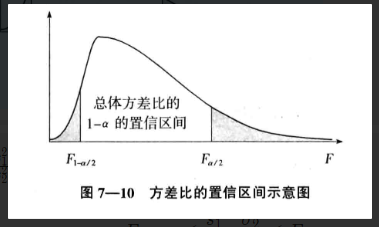📖 由于 s 1 2 s 2 2 ⋅ σ 2 2 σ 1 2 ∼ F ( n 1 − 1 , n 2 − 1 ) \frac{s_1^2}{s_2^2}\cdot\frac{\sigma_2^2}{\sigma_1^2} \sim{F(n_1-1,n_2-1)} ，故可以用它来代替F，公式为：

F 1 − α / 2 ≤ s 1 2 s 2 2 ⋅ σ 2 2 σ 1 2 ≤ F α / 2 F_{1-\alpha/2} \le{\frac{s_1^2}{s_2^2}\cdot\frac{\sigma_2^2}{\sigma_1^2}} \le{F_{\alpha/2}}

🔑 由上式可得，两个总体方差比在 1 − α 1-\alpha 置信水平下的置信区间为：

s 1 2 / s 2 2 F α / 2 ≤ σ 1 2 σ 2 2 ≤ s 1 2 / s 2 2 F 1 − α / 2 \frac{s_1^2/s_2^2}{F_{\alpha/2}} \le{\frac{\sigma_1^2}{\sigma_2^2}} \le{\frac{s_1^2/s_2^2}{F_{1-\alpha/2}}}

其中， F α / 2 F_{\alpha/2} F 1 − α / 2 F_{1-\alpha/2} 是分子自由度为 ( n 1 − 1 ) (n_1-1) 和分母自由度为 ( n 2 − 1 ) (n_2-1) 的F分布的右侧面积为 α / 2 \alpha/2 1 − α / 2 1-\alpha/2 的分位数。

可以利用F分布求得 F 1 − α / 2 F_{1-\alpha/2} ，公式为：

F 1 − α / 2 ( n 1 , n 2 ) = 1 F α ( n 2 , n 1 ) F_{1-\alpha/2}(n_1,n_2)=\frac{1}{F_{\alpha}(n_2,n_1)}

n 1 n_1 表示分子自由度； n 2 n_2 表示分母自由度

# 7.4 如何确定总体估计时需要的样本量？

### 7.4.1 估计总体均值时样本量的确定

📖总体均值的置信区间由样本均值和估计误差两部分组成。

在重复抽样，或无限总体抽样条件下，估计误差为 z α / 2 σ n z_{\alpha/2}\frac{\sigma}{\sqrt{n}}
其中 z α / 2 z_{\alpha/2} 的值和样本量n共同确定了估计误差的大小。
当确定 1 − α 1-\alpha 时， z α / 2 z_{\alpha/2} 就可以确定。

🔑如果给定 z α / 2 z_{\alpha/2} 和总体标准差 σ \sigma ，就可以求得任一指定估计误差所需要的样本量，公式如下：

n = ( z α / 2 ) 2 σ 2 E 2 n=\frac{ (z_{\alpha/2})^2\sigma^2 }{ E^2 }

其中E代表所希望达到的估计误差。

如果 σ \sigma 未知，可以用样本的标准差来代替；也可以用试验调查的办法，选择一个初始样本，以该样本的标准差作为 σ \sigma 的估计值。

📖 样本量与置信水平成正比，置信水平越大，所需的样本量也就越大；
样本量与总体方差成正比，总体的差异越大，要求的样本量也越大；
样本量与估计误差的平方成反比，即可以接受的估计误差的平方越大，所需的样本量越小。

根据公式计算出来的样本数为非整数时，通常取成较大的整数，即样本量的圆整法则。

### 7.4.2 估计总体比例时样本量的确定

📖总体均值的置信区间由样本均值和估计误差两部分组成。

在重复抽样，或无限总体抽样条件下，估计误差为 z α / 2 π ( 1 − π ) n z_{\alpha/2} \sqrt{ \frac{\pi(1-\pi)}{n} }
其中 z α / 2 z_{\alpha/2} 的值、总体比例 π \pi 、样本量n共同确定了估计误差的大小。
当确定 1 − α 1-\alpha 时， z α / 2 z_{\alpha/2} 就可以确定。
总体比例的值是固定的，所以估计误差由样本来确定，样本量越大，估计误差就越小，估计的精度越好。

🔑 如果给定 z α / 2 z_{\alpha/2} 和总体标准差 σ \sigma ，就可以求得任一指定估计误差所需要的样本量，公式如下：

n = ( z α / 2 ) 2 π ( 1 − π ) E 2 n=\frac{ (z_{\alpha/2})^2\pi(1-\pi) }{ E^2 }

其中E代表所希望达到的估计误差，大多数情况下，E＜0.10

如果 π \pi 未知，可以用类似样本的比例来代替；也可以用试验调查的办法，选择一个初始样本，以该样本的比例作 π \pi 的估计值。
π \pi 无法知道时，通常取使 π ( 1 − π ) \pi(1-\pi) 最大时的0.5。

书籍：《统计学（第六版）》
书籍作者：贾俊平

思维导图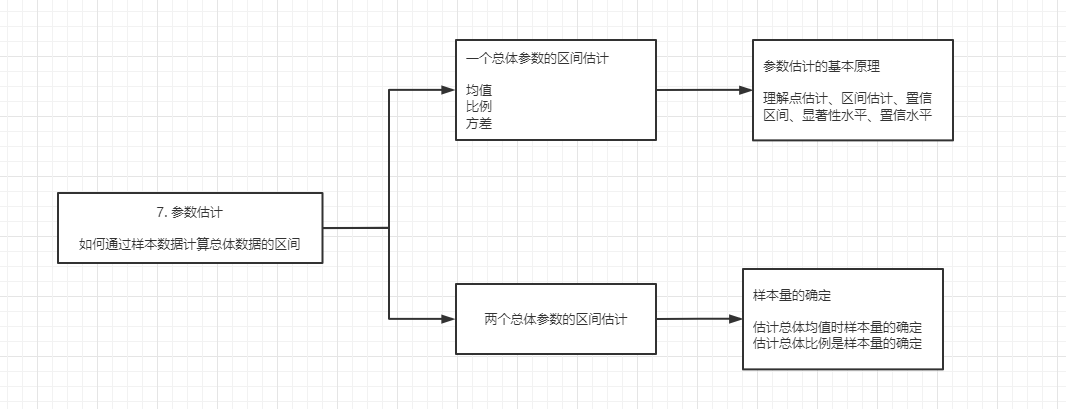展开全文• ## 用样本估计总体

万次阅读 2019-07-04 09:59:07
样本是从总体X中按一定的规则抽出的个体的全部，X1，X2，…，XnX_1，X_2，…，X_nX1​，X2​，…，Xn​表示； 样本中所含个体的个数称为样本容量，nnn表示。 就好比要研究一个班的平均身高: 这个班的所有同学的...
• 设两个总体服从二项分布，这两个总体中具有某种特征单位数的比例分别为π1和π2，但π1和π2未知，可以样本比例p1和p2代替，有两种情况    a、检验两个总体比例相等的假设 设假设的...
• 1. MLE的意义：样本估计总体分布参数 假定一个事件的观测样本服从如下分布，我们如何确定总体数据的分布模型？ 首先应该想到是建立线性回归模型，然而由于该变量不是正态分布的，而且是不对称的，因此不符合线性...
• 参数检验：若样本所来自的总体为分布已知的数学形式（如正态分布），对其总体参数进行假设检验，则称为参数检验。 参数检验的特点： 分析目的：对总体参数(μ π)进行估计或检验。 分 布：要求总体分布已知，如：...统计分析 参数检验
• 没有总体数据，样本3.统计参数2.1.2 指数分布1.有总体数据2.没有总体数据，样本3.统计参数2.2 单样本t检验2.2.1 单样本t检验目的2.2.2 SPSS操作2.3 两独立样本t检验2.3.1 目的2.3.2 SPSS操作2.4 两配对样本t检验...数据挖掘 参数检验
• 如何利用最大似然估计，推演出总体参数最大可能的概率？ 二、对最大似然估计法的详细讲解： 最大似然估计(Maximum Likelihood Estimate) 就是利用已知的样本结果，反推总体参数最大可能的概率。 例如： X~B(n,p)表示...大数据 python 机器学习 人工智能
•  b、置信区间概念图来表示  c、得 到总体均值在μ在1-a置信水平下的置信区间，它由估计值给估计误差组成 置信下限  置信上限  a是事先确定好的...
• ## 推断统计：参数估计和假设检验

千次阅读 多人点赞 2020-03-03 00:35:24
2）为什么要进行推断统计？   3、参数估计(点估计和区间估计)    1）参数估计、点估计和区间统计的概念    2）点估计说明    3）区间估计说明   4、中心极限定理    1）中心极限定理的概念  ...
• 参数估计的MATLAB实现》由会员分享，可在线阅读，更多相关《参数估计的MATLAB实现(17页珍藏版)》请在人人文库网上搜索。1、1,参数估计MATLAB实现,点估计,区间估计,2,点估计,区间估计,矩估计,最大似然估计,参数估计...
• 1、概述 总体、个体、样本 2、点估计和区间估计 2.1、点估计 2.2、区间估计 置信度：总体参数有多大的概率位于置信区间 置信区间：
• 参数估计：通过统计样本估计总体参数总体均值估计：推断总体均值μ的统计量就是样本均值 u, 研究两个总体时，所关心的参数主要是两个总体均值之差（u1 - u2） ； 两个样本是从两个独立总体抽取，两个样本元素...
• ## 总体参数估计概述

千次阅读 2009-10-26 19:47:00
统计推断(Statistical inference)就是根据样本的实际数据，对总体的数量特征作出具有一定可靠程度的估计和判断。统计推断的基本内容有参数估计和假设检验两方面。概括地说，研究一个随机变量，推断它具有什么样的...
• ## 浅谈参数估计

万次阅读 多人点赞 2019-09-03 09:38:34
参数估计是推断统计的重要内容之一,它是在抽样及抽样分布的基础上,根据样本统计量来推断所关心的总体参数. 估计量 如果能够掌握总体的全部数据,那么只需要作一些简单的统计描述,就可以得到所关心的总体特征，比如，...
• 我们希望抽取的样本分布和总体分布一致，这样样本估计总体会比较准确，这种样本叫作无偏样本。 无偏样本的抽样方法： ① 简单随机抽样：抽签，随机编号生成器，有放回抽样和不放回两种 ② 分层抽样：将总体分为几...
• 7.1 参数估计的基本原理 7.1.1 估计量与估计值 1.估计量：用于估计总体参数的随机变量，如样本均值、样本比例、样本方差等 ...1）样本的估计量直接作为总体参数的估计值 · 例如：样本均值直接作为总体...
• ## 参数估计（点估计和区间估计）

万次阅读 多人点赞 2019-09-06 12:07:06
1.点估计就是样本统计量来估计总体参数。 概念理解：当我们想知道某一总体的某个指标的情况时，测量整体该指标的数值 的工作量太大，或者不符合实际，这时我们可以采用抽样的方法选取一部分样本测量出他们数值，...
• 展开全部 英飞凌 印记...对于几何变量，人们含有字母的代数式来表示变量，这个代数式叫作参数式，其中的字母叫做参数图形几何性质与代数关系来连立整式，进而解题。同时“参数法 ”也是许许多多解题技巧的源泉。
• ## 统计学——参数估计与假设检验

万次阅读 多人点赞 2019-02-13 17:15:04
在参数估计中，用来估计总体参数的统计量称为估计量。样本均值、样本比例、样本方差等都可以是一个估计量。而根据一个具体的样本计算出来的估计量的数值称为估计值。 参数估计的方法有点估计和区间估计两种。点估计...机器学习理论基础
• 0x1：数理统计中为什么要引入总体和个体这个概念 概率论与数理统计中，一个很重要的研究对象就是总体的概率分布，理论上说，我们希望获得被研究对象的总体样本，基于这份总体样本进一步研究其概率分布，但是遗憾地...
• 重复抽样：从总体中抽取一个元素后，把这个元素放回到总体中再抽取第二个元素，直至抽取ｎ个元素为止 不重复抽样：一个元素被抽中后不再放回总体，然后再从剩下的元素中抽取第二个元素，直到抽取ｎ个元素为止 二、...
• 我们之前比较两总体均值时，都要假定感兴趣的总体是近似正态分布。然而，在许多情况，这种正态总体的假定是不可靠的。 能否在总体分布不知道的时候有办法检验两个总体的中位数是否相等呢？ 我们在这里，介绍一种常用...R语言
• 匿名用户1级2010-12-28 回答统计学中把总体的指标统称为参数。而由样本算得的相应的总体指标称为统计量。参数一般是确定但未知的，统计量是变化但可知的。统计量统计量是统计理论中用来对数据进行分析、检验的变量。...
• 正态总体参数的检验  1 总体标准差已知时的单个正态总体均值的U检验  例：  某切割机正常工作时，切割的金属棒的长度服从正态分布N(100,4)。从该切割机切割的一批金属棒中随机抽取15根，测得长度为：  97 102 ...
• 1、什么参数估计 简单来说是：参数估计是指使用样本统计量估计总体参数的 【百度百科的解释如下】 参数估计（parameter estimation），统计推断的一种。根据从总体中抽取的随机样本来估计总体分布中未知参数的...
• ## 非参数估计：核密度估计KDE

万次阅读 多人点赞 2016-12-14 11:38:46
http://blog.csdn.net/pipisorry/article/details/53635895核密度估计Kernel Density Estimation(KDE)概述密度估计的问题...参数估计参数估计又可分为参数回归分析和参数判别分析。在参数回归分析中，人们假定数据分布kde 非参数估计
• ## 非参数统计

千次阅读 2019-12-18 15:44:42
然而，在实际问题中也有这样的情况：对样本分布族并未给出其数学形式，而只做了某些一般的假定，如只假定总体分布的连续性或对称性，而对其分布的数学形式则一无所知。类似这种情形的统计问题称为非参数型的。 如果......